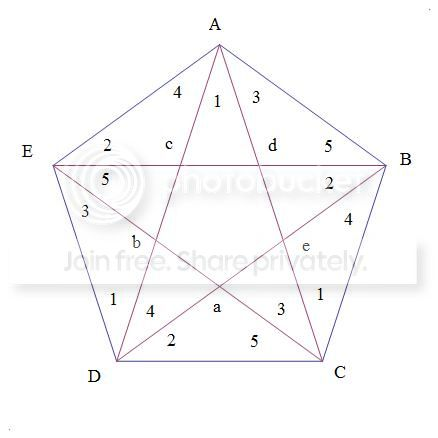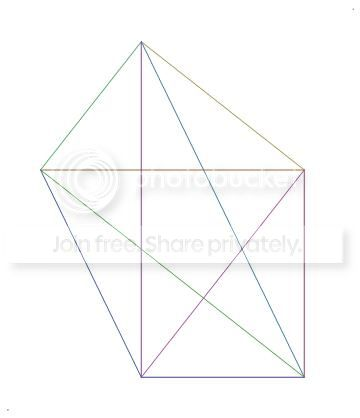# Pentagon with equal-area Triangles

Many of you have come across a certain type of pentagon:

Let us label a pentagon $ABCDE$. It satisfies that $[ABC]=[BCD]=[CDE]=[DEA]=[EAB]$ where $[XYZ]$ means the area of $\triangle XYZ$.

The question: What is the least amount of obtuse angles the pentagon must have? Prove your claim.Note by Daniel Liu
7 years ago

This discussion board is a place to discuss our Daily Challenges and the math and science related to those challenges. Explanations are more than just a solution — they should explain the steps and thinking strategies that you used to obtain the solution. Comments should further the discussion of math and science.

When posting on Brilliant:

• Use the emojis to react to an explanation, whether you're congratulating a job well done , or just really confused .
• Ask specific questions about the challenge or the steps in somebody's explanation. Well-posed questions can add a lot to the discussion, but posting "I don't understand!" doesn't help anyone.
• Try to contribute something new to the discussion, whether it is an extension, generalization or other idea related to the challenge.

MarkdownAppears as
*italics* or _italics_ italics
**bold** or __bold__ bold
- bulleted- list
• bulleted
• list
1. numbered2. list
1. numbered
2. list
Note: you must add a full line of space before and after lists for them to show up correctly
paragraph 1paragraph 2

paragraph 1

paragraph 2

[example link](https://brilliant.org)example link
> This is a quote
This is a quote
    # I indented these lines
# 4 spaces, and now they show
# up as a code block.

print "hello world"
# I indented these lines
# 4 spaces, and now they show
# up as a code block.

print "hello world"
MathAppears as
Remember to wrap math in $$ ... $$ or $ ... $ to ensure proper formatting.
2 \times 3 $2 \times 3$
2^{34} $2^{34}$
a_{i-1} $a_{i-1}$
\frac{2}{3} $\frac{2}{3}$
\sqrt{2} $\sqrt{2}$
\sum_{i=1}^3 $\sum_{i=1}^3$
\sin \theta $\sin \theta$
\boxed{123} $\boxed{123}$

Sort by:Pentagon

For all those triangles to have equal areas, then all the chords must be parallel to the opposite side. From this, all the angles ${1, 2, 3, 4, 5}$ must be as shown in the figure above. Now, given a vertex, say, $A$, which is the sum of angles ${4, 1, 3}$, for this to be obtuse, then angle $a$ must be $<90$. If this is so, then angles $b$ and $e$ cannot be $<90$. If one of the remaining angles $b, c$ is $<90$, then the other cannot be. Thus, at most, only $2$ vertices can be acute, which means at least $3$ of the triangles must be obtuse.

I'm not sure if this is what is to be proven, but certainly no triangle can have more than one obtuse angle.

- 7 years ago

Sorry, typoed! I wanted to least amount of obtuse angles the pentagon could have.

- 7 years ago

Well, fix it, Daniel. It was a fun problem, once I decided what the problem was. See my motto. Maybe later I'll put up a graphic of such a pentagon with just 3 obtuse angles.

- 7 years ago

Huh, I fixed it when I commented. Must have been a glitch or something. Fixed it again.

- 7 years ago

Here's the case where such a pentagon has 2 right angle verticesRight Angles Pentagon

In working this one out, once one vertex was made a right angle, it works out that there is only one degree of freedom to adjust the angle of the other vertex to make it a right angle, so that making any more vertices a right angle was not possible. As per the proof given above.

The figure given here has a lot of interesting proportions.

- 7 years ago

If I may ask, how do you post pictures here?

- 7 years ago

I picked this up while working on Brilliant. To post a picture, enter the following Latex coding

!$[...title... ]$ $(...http...address...)$

- 6 years, 12 months agoPicture

OK I got it! Thanks!!

- 6 years, 12 months ago

I have found a way to construct such a pentagon which has NO obtuse angles: http://i60.tinypic.com/2yocn4w.png

This pentagon has 3 acute angles and 2 reflex angles, none of which are obtuse (an obtuse angle is an angle $\theta$ such that $90 < \theta < 180$).

In the diagram, let $\angle BAE = \angle ABC = x = 30$, $\angle CDE = 2y = arcsin \left(\frac{\sqrt{3}}{4}\right)$, $z = 90+x+y$, $AB = 1$, $AE = BC = m = \frac{sin(x)sin(2y)}{cos^2 (x+y)}$, and $CD = DE = n = \frac{sin(x)}{cos(x+y)}$.

By symmetry we see that $[ABC] = [EAB]$ and $[DEA] = [BCD]$.

Now, we see that $[EAB] = \frac{m*1*sin(x)}{2}$ and, by definition of $z$ and $n$, we have:

$[DEA] = \frac{m*n*sin(z)}{2} = \frac{m*n*sin(90+x+y)}{2} = \frac{m*(n*cos(x+y))}{2} = \frac{m*sin(x)}{2} = [EAB]$

Thus $[DEA] = [EAB]$.

Now by definition of $m$, $n$ and $z$, notice that $m*sin(z) = m*cos(x+y) = \frac{sin(x)sin(2y)}{cos(x+y)} = n*sin(2y)$. Therefore,

$[DEA] = \frac{n*(m*sin(z))}{2} = \frac{n*(n*sin(2y))}{2} = [CDE]$

Therefore, $[ABC] = [BCD] = [CDE] = [DEA] = [EAB]$. Finally we need to show that this pentagon is "anatomically correct" - i.e. that the defined side lengths and angles create a correct pentagon. To do this, we simply need to show that $m*cos(x) + n*sin(y) = \frac{1}{2}$ (so that the horizontal components match up correctly).

$m*cos(x) + n*sin(y) = \frac{sin(x)cos(x)sin(2y) + sin(x)sin(y)cos(x+y)}{cos^2(x+y)}$

$= \frac{\frac{1}{2} * \frac{\sqrt{3}}{2} * \frac{\sqrt{3}}{4} + \frac{1}{2}sin(y)cos(30+y)}{cos^2(30+y)}$

$= \frac{3 + 8sin(y) \left[\frac{\sqrt 3}{2} cos(y) - \frac{1}{2} sin(y) \right]}{16 \left[\frac{\sqrt{3}}{2} cos(y) - \frac{1}{2} sin(y) \right]^2}$

$= \frac{3 + 2\sqrt 3 sin(2y) - 4sin^2(y)}{12cos^2(y) - 8 \sqrt 3 cos(y)sin(y) + 4sin^2(y)}$

$= \frac{3 + \frac{3}{2} - 4sin^2(y)}{12 - 4 \sqrt 3 sin(2y) - 8sin^2(y)}$

$= \frac{\frac{9}{2} - 4sin^2(y)}{9 - 8sin^2(y)} = \frac{1}{2}$

Therefore, this pentagon satisfies the necessary conditions and has no obtuse angles. Therefore the least possible amount of obtuse angles is zero.

- 7 years ago

Ah, I assumed that the pentagon was convex. Nice job finding a concave pentagon with no obtuse angles though.

- 7 years ago

Well, the question didn't specify :P Thanks!

- 7 years ago

That would work, given that the technical definition of an "obtuse angle" is between 90 and 180 degrees, and that the angle of a vertex in any closed polygon, convex or not, is measured on the inside.

- 7 years ago